# ECI Gaussian Indicator for ThinkorSwim#### horserider

##### Well-known member
VIP
Did not find this in a search. Sorry if it already was posted.

It is a compression study is very fast at showing compression. Has some hints of when compression turns into expansion.

Code:
``````#hint: Expansion Contraction Indicator (ECI): \n ECI Identifies areas of price compression which leads to price expansion. ECI doesn't show the direction of a trend but does indicate when a trend is going to resume (price flagging) or if a change in trend polarity is happening.\n ECI plots the areas where price becomes compressed with colored and clouded bands. The indicator also leaves legacy points in areas of past compression.
# ECI is based on the FE algorithm
# Original plot style by Mobius
# Note: Default Lengths are not optimized. My personal experience has been length ranges between 8 and 13 are generally effective.
# Here's another way to look at Fractal Energy. This study plots it on the chart graph with clouded areas for the current price compression, exhaustion area and leaves colored points as a legacy plot for past areas.
# Good CSA choices with this study are:
# ECI + SuperTrend + Fractal Pivots
# ECI + SuperTrend + RSI in Laguerre Time
# ECI + SuperTrend + Standard Deviation Bands
# ECI + Linear Regression Standard Deviation Bands
# This version Modified to filter price to a normalized distribution

# User Inputs
input EciLength = 14; #hint EciLength: Length for calculations.
input AvgLength = 10; #hint AvgLength: Length for smoothing.
input AvgType = AverageType.Simple; #hint AvgType: Average Type
input MeanValue = HL2; #hint MeanValue: Point of origen.
input DisplayPoints = yes; #hint DisplayPoints: No Points.
input OnExpansion = yes; #hint OnExpansion: Line extensions.

# Variables
script g {
input data = close;
def w = (2 * Double.Pi / 8);
def beta = (1 - Cos(w)) / (Power(1.414, 2.0 / 4) - 1 );
def alpha = (-beta + Sqrt(beta * beta + 2 * beta));
def G = Power(alpha, 4) * data +
4 * (1 – alpha) * G – 6 * Power( 1 - alpha, 2 ) * G +
4 * Power( 1 - alpha, 3 ) * G - Power( 1 - alpha, 4 ) * G;
plot Line = G;
}
def o = g(data = open);
def h = g(data = high);
def l = g(data = low);
def c = g(data = close);
def bar = BarNumber();
def HMax = Highest(Max(h, c), EciLength);
def LMax = Lowest(Min(l, c), EciLength);
def TR = HMax - LMax;
def ECI = Round((Log(Sum(TrueRange(h, c, l), EciLength) / TR) /
Log(EciLength)) / TickSize(), 0) * TickSize();
def Avg = MovingAverage(AverageType = AvgType, ECI, AvgLength);
def S1 = if ECI crosses above Avg
then MeanValue
else S1;
def S = ECI > Avg;
def SBars = if ECI > Avg
then bar
else Double.NaN;
def StartBar = if ECI crosses above Avg
then bar
else StartBar;
def LastSBar = if ECI crosses below Avg
then bar
else LastSBar;
def PP = if ECI crosses above Avg
then MeanValue
else PP;
def Mean_Limit = if bar != StartBar
then bar - StartBar
else if bar == StartBar
then Double.NaN
else Mean_Limit;
def SHigh = if ECI crosses above Avg
then h
else SHigh;
def SHighBar = if S and
h == SHigh
then bar
else SHighBar;
def SHigh_Limit = if bar == StartBar
then Double.NaN
else if bar > StartBar
then bar - SHighBar
else SHigh_Limit;
def SLow = if ECI crosses above Avg
then l
else SLow;
def SLowBar = if S and
l == SLow
then bar
else SLowBar;
def SLow_Limit = if bar == StartBar
then Double.NaN
else if bar > StartBar
then bar - SLowBar
else SLow_Limit;
# Internal Script Reference
script LinePlot {
input LineLimit = 0;
input OnExpansion = yes;
input data = close;
input bar = 0;
def ThisBar = HighestAll(bar);
def cLine = if bar == ThisBar
then data
else Double.NaN;
def cond1 = CompoundValue(1, if IsNaN(data)
then cond1
else data, data);
plot P = if ThisBar - LineLimit <= bar
then HighestAll(cLine)
else Double.NaN;
plot ExpLine = if OnExpansion and
IsNaN(data[-1])
then cond1
else Double.NaN;
}
# Plots
plot SD_Pivot = LinePlot(data = PP, LineLimit = Mean_Limit, OnExpansion = OnExpansion, bar = StartBar).P;
plot SD_Pivot_X = LinePlot(data = PP, LineLimit = StartBar).ExpLine;
SD_Pivot.SetDefaultColor(Color.CYAN);
SD_Pivot_X.SetDefaultColor(Color.CYAN);
plot SD_R1 = LinePlot(data = SHigh, LineLimit = SHigh_Limit, OnExpansion = OnExpansion, bar = SHighBar).P;
plot SD_R1_X = LinePlot(data = SHigh, LineLimit = SHigh_Limit).ExpLine;
SD_R1.SetDefaultColor(Color.Light_GREEN);
SD_R1_X.SetDefaultColor(Color.Light_GREEN);
plot SD_S1 = LinePlot(data = SLow, LineLimit = SLow_Limit, OnExpansion = OnExpansion, bar = SLowBar).P;
plot SD_S1_X = LinePlot(data = SLow, LineLimit = SLow_Limit).ExpLine;
SD_S1.SetDefaultColor(Color.Light_RED);
SD_S1_X.SetDefaultColor(Color.Light_RED);
plot SPlot = if S
then S1 #l - (2 * TickSize())
else Double.NaN;
SPlot.SetHiding(!DisplayPoints);
SPlot.SetPaintingStrategy(PaintingStrategy.POINTS);
SPlot.SetLineWeight(1);
SPlot.SetDefaultColor(Color.Yellow);
AddLabel(1, "Energy Level = " + ECI, color.white);
# End Code Modified ECI``````

Last edited by a moderator:#### horserider

##### Well-known member
VIP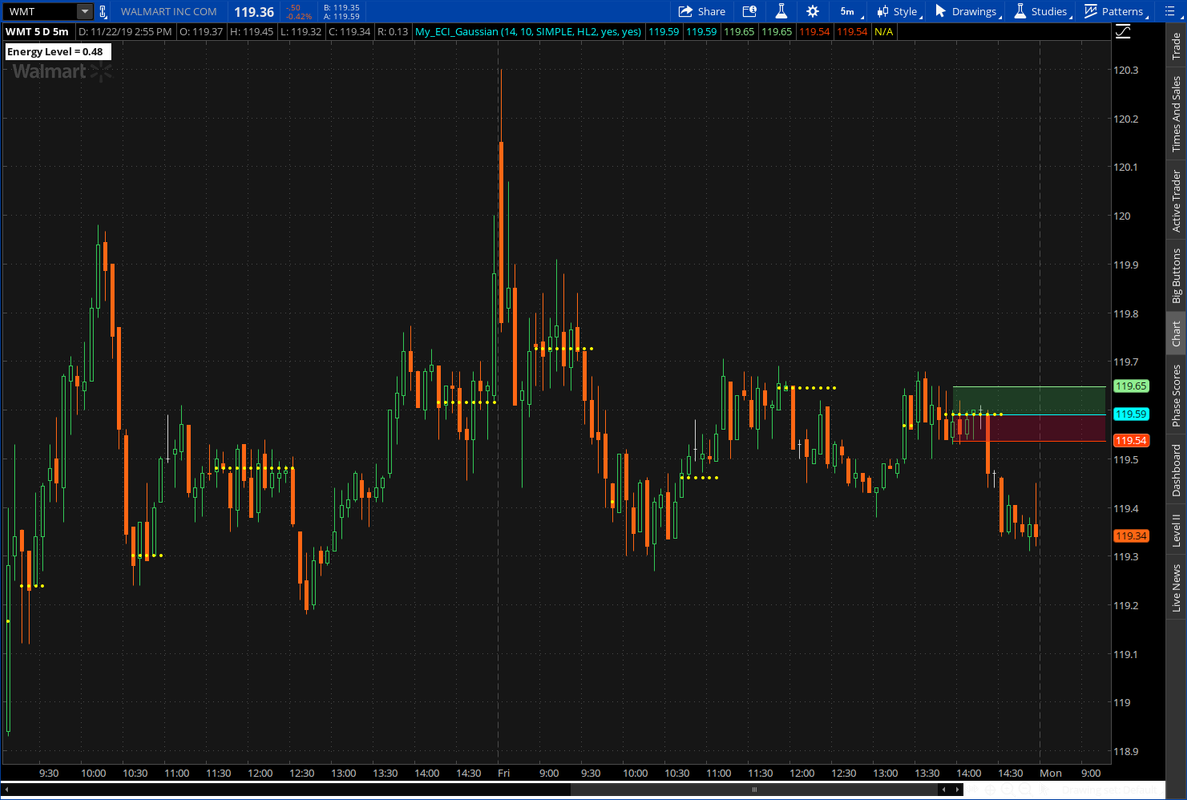•markos
T

#### tomsk

##### Well-known member
VIP
Given some of the recent discussion on consolidation and Mobius ECI study, for those folks that might be interested to scan the universe of stocks for such setups, I have converted Mobius ECI study into a scan. Two scan conditions are created - one for the bullish case and another for the bearish case. I have also removed extraneous variables not required for the scan.

Tested this on a scan of the S&P 500 (Daily aggregation) with the following results
Bullish scan - 138 hits, e.g. AMZN
Bearish scan - 20 hits e.go. PSX

Please remember that the scanner only expects one plot so comment out the scan plot statement you do not wish.
Here then is the scan code

Code:
``````# ECI Scan
# tomsk
# 11.25.2019

# Given some of the recent discussion on consolidation and Mobius ECI study,
# for those folks that might be interested to scan the universe of stocks for
# such setups, I have converted Mobius ECI study into a scan. Two scan conditions
# are created - one for the bullish case and another for the bearish case. I
# have also removed variables not required for the scan.

# Expansion Contraction Indication
# Flags: Uses Fractal enregy to locate price compression or flag areas
# Mobius
# 3.18.2017

# User Inputs
input EciLength = 5; #hint EciLength: Length for calculations.
input AvgType = AverageType.Simple; #hint AvgType: Average Type
input MeanValue = HL2; #hint MeanValue: Point of origen.

script G {
input p = close;
input n = 2;
input b = 1.05;
def w;
def beta;
def alpha;
def G;
w = (2 * Double.Pi / n);
beta = (1 - Cos(w)) / (Power(1.414, 2.0 / b) - 1 );
alpha = (-beta + Sqrt(beta * beta + 2 * beta));
G = Power(alpha, 4) * p +
4 * (1 – alpha) * G – 6 * Power( 1 - alpha, 2 ) * G +
4 * Power( 1 - alpha, 3 ) * G - Power( 1 - alpha, 4 ) * G;
plot data = G;
}
# Variables:
def bar = barNumber();
def o = g(open, ECIlength, betaDev);
def h = g(high, ECIlength, betaDev);
def l = g(low, ECIlength, betaDev);
def c = g(close, ECIlength, betaDev);
def ECI = Log(Sum(Max(h, c) - Min(l, c), ECIlength) /
(Highest(h, ECIlength) - Lowest(l, ECIlength))) /
Log(ECIlength);
def Avg = MovingAverage(AverageType = AvgType, ECI, ECILength);
def S1 = if ECI crosses above Avg
then MeanValue
else S1;
def S = ECI > Avg;
def SBars = if ECI > Avg
then bar
else Double.NaN;
def StartBar = if ECI crosses above Avg
then bar
else StartBar;
def LastSBar = if ECI crosses below Avg
then bar
else LastSBar;
def PP = if ECI crosses above Avg
then MeanValue
else PP;
def Mean_Limit = if bar != StartBar
then bar - StartBar
else if bar == StartBar
then Double.NaN
else Mean_Limit;
def SHigh = if ECI crosses above Avg
then h
else SHigh;
def SHighBar = if S and
h == SHigh
then bar
else SHighBar;
def SHigh_Limit = if bar == StartBar
then Double.NaN
else if bar > StartBar
then bar - SHighBar
else SHigh_Limit;
def SLow = if ECI crosses above Avg
then l
else SLow;
def SLowBar = if S and
l == SLow
then bar
else SLowBar;
def SLow_Limit = if bar == StartBar
then Double.NaN
else if bar > StartBar
then bar - SLowBar
else SLow_Limit;

# Comment out (#) the ONE not needed
plot BullScan = close crosses above SHigh;
#plot BearScan = close crosses below SLow;
# ECI Scan``````

••MerryDay, J007RMC and BenTen
M

#### mmm777

##### New member
2019 Donor
thanks. it is very good indicator , but scanner is just for uptrend, can you make one for downtrend.

C

#### chillc15

##### New member
@mmm777

Per @tomsk you have to comment out which one you don't want.

# Comment out (#) the ONE not needed
plot BullScan = close crosses above SHigh;
#plot BearScan = close crosses below SLow;
# ECI Scan

# Comment out (#) the ONE not needed
#plot BullScan = close crosses above SHigh;
plot BearScan = close crosses below SLow;
# ECI Scan

Last edited by a moderator:
H

#### HighBredCloud

##### Well-known member
VIP
@chillc15 so for the rest of the none coders on this site...how is that suppose to look like? I know it easy for most of you...but where exactly do you comment out? What part?#### BenTen

Staff
VIP
@HighBredCloud The code posted by @chillc15 already commented out the stuff for you.

Take a look at the first portion:

Code:
``````# Comment out (#) the ONE not needed
plot BullScan = close crosses above SHigh;
#plot BearScan = close crosses below SLow;
# ECI Scan``````

Notice how in front of plot BullScan there isn't a # sign? That means you're looking for bullish signals. Now, if you want to scan for bearish signals, then add # back to the BullScan and remove the # from plot BearScan.

••vro3, RickAns, markos and 2 others
H

#### HighBredCloud

##### Well-known member
VIP
@BenTen OK...so it looks like just the bottom of the code needs to be changed with the # mark in order to see which scan you want...

I had no idea where to insert the portion @chillc15 did...Thank You!

T

#### tomsk

##### Well-known member
VIP
Just to be sure that EVERYONE understands how to interpret this, at the end of the scan there is a section of code like so:

# Comment out (#) the ONE not needed
plot BullScan = close crosses above SHigh;
#plot BearScan = close crosses below SLow;

Notice that there are some lines that are commented out with (#) - Those would be ignored by the scanner, only for human consumption
In the above example only the bullish scan is enabled.

In order to turn this into a bearish scan, just switch the commented lines like so

# Comment out (#) the ONE not needed
#plot BullScan = close crosses above SHigh;
plot BearScan = close crosses below SLow;

Compare those differences - it really is that simple.
Just REMEMBER that there can only be one active plot defined or the scanner would complain

OK Folks?

@BenTen If there is not already a tutorial that covers scan specifics, perhaps you can add this commentary there for any future folks who may require a little bit more assistance

•RickAns, mmm777 and BenTen
D

#### diazlaz

##### Well-known member
2019 Donor
VIP
Hi everyone, if the ECI is trend is quite interesting, amazing works well with lower and higher time frames. any ideas if a MTF version of this would yield any value? thoughts?

T

#### tomsk

##### Well-known member
VIP
Most folks use MTF studies to determine trend, momentum, breadth, sentiment across different time frames to determine if they have an edge to undertake trading decisions. The ECI is a bit different. The concept of the ECI is very simple - identifies areas of price compression which in turn leads to price expansion. It doesn't show the direction of a trend but indicates when a trend is going to resume or if there is a change in polarity of the trend.

•diazlaz#### J007RMC

##### Well-known member
2019 Donor
VIP
Given some of the recent discussion on consolidation and Mobius ECI study, for those folks that might be interested to scan the universe of stocks for such setups, I have converted Mobius ECI study into a scan. Two scan conditions are created - one for the bullish case and another for the bearish case. I have also removed extraneous variables not required for the scan.

Tested this on a scan of the S&P 500 (Daily aggregation) with the following results
Bullish scan - 138 hits, e.g. AMZN
Bearish scan - 20 hits e.go. PSX

Please remember that the scanner only expects one plot so comment out the scan plot statement you do not wish.
Here then is the scan code

Code:
``````# ECI Scan
# tomsk
# 11.25.2019

# Given some of the recent discussion on consolidation and Mobius ECI study,
# for those folks that might be interested to scan the universe of stocks for
# such setups, I have converted Mobius ECI study into a scan. Two scan conditions
# are created - one for the bullish case and another for the bearish case. I
# have also removed variables not required for the scan.

# Expansion Contraction Indication
# Flags: Uses Fractal enregy to locate price compression or flag areas
# Mobius
# 3.18.2017

# User Inputs
input EciLength = 5; #hint EciLength: Length for calculations.
input AvgType = AverageType.Simple; #hint AvgType: Average Type
input MeanValue = HL2; #hint MeanValue: Point of origen.

script G {
input p = close;
input n = 2;
input b = 1.05;
def w;
def beta;
def alpha;
def G;
w = (2 * Double.Pi / n);
beta = (1 - Cos(w)) / (Power(1.414, 2.0 / b) - 1 );
alpha = (-beta + Sqrt(beta * beta + 2 * beta));
G = Power(alpha, 4) * p +
4 * (1 – alpha) * G – 6 * Power( 1 - alpha, 2 ) * G +
4 * Power( 1 - alpha, 3 ) * G - Power( 1 - alpha, 4 ) * G;
plot data = G;
}
# Variables:
def bar = barNumber();
def o = g(open, ECIlength, betaDev);
def h = g(high, ECIlength, betaDev);
def l = g(low, ECIlength, betaDev);
def c = g(close, ECIlength, betaDev);
def ECI = Log(Sum(Max(h, c) - Min(l, c), ECIlength) /
(Highest(h, ECIlength) - Lowest(l, ECIlength))) /
Log(ECIlength);
def Avg = MovingAverage(AverageType = AvgType, ECI, ECILength);
def S1 = if ECI crosses above Avg
then MeanValue
else S1;
def S = ECI > Avg;
def SBars = if ECI > Avg
then bar
else Double.NaN;
def StartBar = if ECI crosses above Avg
then bar
else StartBar;
def LastSBar = if ECI crosses below Avg
then bar
else LastSBar;
def PP = if ECI crosses above Avg
then MeanValue
else PP;
def Mean_Limit = if bar != StartBar
then bar - StartBar
else if bar == StartBar
then Double.NaN
else Mean_Limit;
def SHigh = if ECI crosses above Avg
then h
else SHigh;
def SHighBar = if S and
h == SHigh
then bar
else SHighBar;
def SHigh_Limit = if bar == StartBar
then Double.NaN
else if bar > StartBar
then bar - SHighBar
else SHigh_Limit;
def SLow = if ECI crosses above Avg
then l
else SLow;
def SLowBar = if S and
l == SLow
then bar
else SLowBar;
def SLow_Limit = if bar == StartBar
then Double.NaN
else if bar > StartBar
then bar - SLowBar
else SLow_Limit;

# Comment out (#) the ONE not needed
plot BullScan = close crosses above SHigh;
#plot BearScan = close crosses below SLow;
# ECI Scan,,,``````

I ran this but added a few scan parameters that returned 6 stocks FRI. needless to say all very bullish.
ECI Bull Scan/ set daily
min \$20.00
% change plus min 2% max 10%
avg vol 30 simple up to 1 mil

•MerryDay and markos
K

#### kelvin

##### Member
VIP
very nice idea, thanks

•markos
T

##### Active member
2019 Donor
Just to be sure that EVERYONE understands how to interpret this, at the end of the scan there is a section of code like so:

# Comment out (#) the ONE not needed
plot BullScan = close crosses above SHigh;
#plot BearScan = close crosses below SLow;

Notice that there are some lines that are commented out with (#) - Those would be ignored by the scanner, only for human consumption
In the above example only the bullish scan is enabled.

In order to turn this into a bearish scan, just switch the commented lines like so

# Comment out (#) the ONE not needed
#plot BullScan = close crosses above SHigh;
plot BearScan = close crosses below SLow;

Compare those differences - it really is that simple.
Just REMEMBER that there can only be one active plot defined or the scanner would complain

OK Folks?

@BenTen If there is not already a tutorial that covers scan specifics, perhaps you can add this commentary there for any future folks who may require a little bit more assistance
Question, making sure I understand this, can you have the bull scan and a bear scan in the same code or do you have to have two different quote boards one for the bull scan and another one for the bear? thanks

T

#### tomsk

##### Well-known member
VIP
Not sure where the misunderstanding is. The quoted comment you included in the above post refers to a scan. A scan only accepts a single plot statement so that was the reason I mentioned it.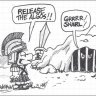#### markos

##### Well-known member
VIP
Question, making sure I understand this, can you have the bull scan and a bear scan in the same code or do you have to have two different quote boards one for the bull scan and another one for the bear? thanks
@Trading51 As @tomsk said, you can only have one plot in a scan.
Thus, if you want a bullish scan, you put a Hashtag, Pound Sign, Comment (all the same thing >>> #) in front of the line of code that you do not want, which is the "plot bear scan" code.

Commenting out a line or 2 of code saves you from deleting it and then searching for the original to get that code back.Anywhere, at the beginning of any line of code, in any study, scan, marketwatch column or whatever, a person can put this little symbol # in front of any line of code and it will render that line of code unreadable by the computer. (Sorry for yelling)

If a person wants a bull scan and a bear scan, save one as Yogi Bull and the other as Yogi Bear, or whatever one wishes. and call up which ever scan you wish and it should perform as expected.

If all else fails, read the Tutorials Here and on TOS. Thanks!

Last edited:
•#### horserider

##### Well-known member
VIP
@markos Copy/ paste/ save your keyboard.•markos#### markos

##### Well-known member
VIP
@horserider yeah, kind of over did it...

T

#### tomsk

##### Well-known member
VIP
@horserider yeah, kind of over did it...

@markos When in doubt always OVER communicate and that is precisely what you've delivered on. Very well written

•surferdude and BenTen#### horserider

##### Well-known member
VIP
@markos Nope. Great explaination. I was referring to how many times this same question comes up. Would be easier to copy paste your answer.

•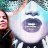ECI High Agg Backtesting Questions 0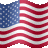Upper Gaussian Mean crossovers with lower macd/gacd Indicators 7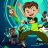Gaussian Rainbow MA Indicator for ThinkorSwim Indicators 1